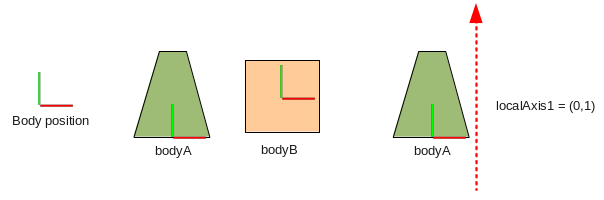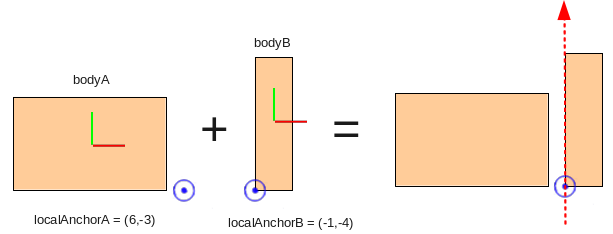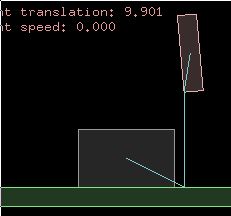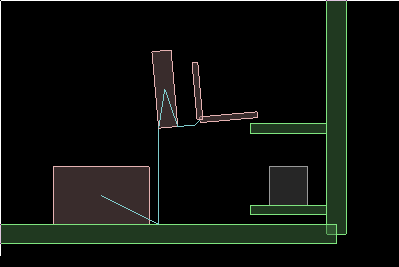## Box2D C++ tutorials - Joints - prismatic

Last edited: July 14 2013

Chinese version -> 中文

### Prismatic joints

The prismatic joint is probably more commonly known as a slider joint. The two joined bodies have their rotation held fixed relative to each other, and they can only move along a specified axis. Prismatic joints can be given limits so that the bodies can only move along the axis within a specific range. They can also be given a motor so that the bodies will try to move at a given speed, with a given force. Common uses for prismatic joints include:
• elevators
• moving platforms
• sliding doors
• pistons

### Creating a prismatic joint

A prismatic joint is created by first setting the desired properties in a b2PrismaticJointDef, then passing that to CreateJoint to get an instance of a b2PrismaticJoint object. We saw in the joints overview that all joint definitions have some properties in common - the two bodies to be joined, and whether they should collide with each other. So we'll set those first:
 ```1 2 3 4 ``` ```  b2PrismaticJointDef prismaticJointDef;   prismaticJointDef.bodyA = bodyA;   prismaticJointDef.bodyB = bodyB;   prismaticJointDef.collideConnected = false;```
Then we come to a bunch of properties which are specific to prismatic joints.
• localAxis1* - the axis (line) of movement (relative to bodyA)
• referenceAngle - the angle to be enforced between the bodies
• localAnchorA - a point in body A to keep on the axis line
• localAnchorB - a point in body B to keep on the axis line
• enableLimit - whether the joint limits will be active
• lowerTranslation - position of the lower limit
• upperTranslation - position of the upper limit
• enableMotor - whether the joint motor will be active
• motorSpeed - the target speed of the joint motor
• maxMotorForce - the maximum allowable force the motor can use
* Changed to localAxisA in Box2D v2.2.0

Let's take a look at what these do in more detail.

As an example of setting up prismatic joints, we'll make a simple forklift. This will make use of joint limits and motors. Here are the bodies we'll use for this - one large box for the chassis and a smaller one for the lift slider. Since we have already covered many examples I will no longer be showing the full listing for all the code, but it's probably convenient to start with a basic 'fenced in' type scene as in some of the previous topics to stop things flying off the screen.
 ```1 2 3 4 5 6 7 8 9 10 11 12 13 14 15 16 17 18 19 20 21 22 23 ``` ```  //body and fixture defs - the common parts   b2BodyDef bodyDef;   bodyDef.type = b2_dynamicBody;   b2FixtureDef fixtureDef;   fixtureDef.density = 1;     //two boxes   b2PolygonShape squareShapeA;   squareShapeA.SetAsBox(5,3);   b2PolygonShape squareShapeB;   squareShapeB.SetAsBox(1,4);     //large box a little to the left   bodyDef.position.Set(-10, 10);   fixtureDef.shape = &squareShapeA;   m_bodyA = m_world->CreateBody( &bodyDef );   m_bodyA->CreateFixture( &fixtureDef );     //smaller box a little to the right   bodyDef.position.Set( -4, 10);   fixtureDef.shape = &squareShapeB;   m_bodyB = m_world->CreateBody( &bodyDef );   m_bodyB->CreateFixture( &fixtureDef );```### Joint axis

The joint axis is the line along which the bodies can move relative to each other. It is specified in bodyA's local coordinates, so you could think of it as the direction bodyB can move from bodyA's point of view. For example if bodyA has a trapezoidal fixture, and bodyB has a square polygon fixture as below, and you want bodyB to be able to slide along the direction the trapezoid is 'pointing'......you would need a local axis of (0,1). So the code for this case would be:
 ```1 ``` `  prismaticJointDef.localAxis1.Set(0,1);`
* Changed to localAxisA in Box2D v2.2.0
This means that as bodyA moves around in the world, the line along which bodyB can slide will move with it, for example:Note that the axis itself is not related to any particular point in the body, it only specifies a direction for the sliding movement. That's why I have intentionally shown it outside the body's fixture in the diagram :) The axis given should be a unit vector, so before you create the joint remember to normalize the vector if it had a length other than 1:
 ```1 ``` `  prismaticJointDef.localAxis1.Normalize();`
Also note that since this only specifies a direction for sliding, the negative of this vector is an equivalent direction, eg. in the example above we could also have used (0,-1). For setting joint limits and motors this will become important.

Let's make the axis for our forklift joint (0,1) so that a positive movement in the axis raises the lift slider.

### Local anchors

Now that we have established the direction along which the two bodies should move with respect to each other, we can specify points on each body that should stay on the axis line. These are given in local coordinates for each body, so you need to remember to look from the point of view of the body in question. Getting back to the forklift example, let's say we want to have the lift slider (bodyB) a little to the right of the main body (bodyA). We could use positions like this:```1 2 ``` ```  prismaticJointDef.localAnchorA.Set( 6,-3);//a little outside the bottom right corner   prismaticJointDef.localAnchorB.Set(-1,-4);//bottom left corner```
Now that we have some sensible values in place for a basic prismatic joint, we can create the joint itself (here I am assuming there is a class variable to store the joint pointer in to access it later).
 ```1 ``` `  m_joint = (b2PrismaticJoint*)m_world->CreateJoint( &prismaticJointDef );`
When you run this you will see if you pause the simulation right at the beginning, the bodies are initially at their defined positions before the prismatic constraint takes effect in the first time step.Since the axis of the joint is (0,1) and none of the bodies are rotated, we could actually have used any old value for the y-value of these anchor points and the bodies would still slide along the same line. However, when we want to set limits for the joint we'll need to be aware of where these points are on the line in order to get the limits in the right place. To make it easier to understand the values for joint limits in the sections below, let's add some output on the screen to show the current joint translation and speed:
 ```1 2 3 4 5 ``` ```  //in Step()   m_debugDraw.DrawString(5, m_textLine, "Current joint translation: %.3f", m_joint->GetJointTranslation());   m_textLine += 15;   m_debugDraw.DrawString(5, m_textLine, "Current joint speed: %.3f", m_joint->GetJointSpeed());   m_textLine += 15;```
Here are a couple of example positions. In the left one, the anchor points we specified are at the same location, so the translation of the joint is considered to be zero. In the right example, the joint anchor of the lift slider (bottom left corner of small body) has moved so that it is about the same height as the top of the large body, which as you'll recall is 6 units high, as confirmed by the on-screen display.If you pick the bodies up and move them around you'll see how the axis is always the same relative to the two bodies, and the translation is only measured along this axis.### Reference angle

The angles of the bodies in this example started at the default angle of zero, and when we constrain them with the prismatic joint they cannot rotate to any other angle (relative to the other body). If we want to have a different angle between the bodies, we can set that with the reference angle property of the prismatic joint definition. The reference angle is given as the angle of bodyB, as seen from bodyA's point of view.

As an example, let's say we want the lift slider body to be tilted back a little to help keep the cargo from falling off the forks. We can give the joint a reference angle of 5 degrees, which will make the joint hold bodyB at 5 degrees counter-clockwise to bodyA.
 ```1 ``` `  prismaticJointDef.referenceAngle = 5 * DEGTORAD;`Note that since the local anchor point of each body is constrained to be on the axis line, the reference angle effectively causes bodyB to rotate around the local anchor point (at the bottom left corner).

### Prismatic joint limits

With the settings made so far the two bodies are free to slide along the line indefinitely, but we can limit this range of motion by specifying limits for the joint. Joint limits define a lower and upper bound on the joint translation within which the bodies will be kept. This is where it comes in handy to have the joint translation displayed on the screen, so we can easily see where the limits should be set by moving the bodies around a bit. For this forklift example, we could set the lower limit at zero (this is where the lift slider body touches the ground) and the upper limit at hmm... about 10 looks fine.
 ```1 2 3 ``` ```  prismaticJointDef.enableLimit = true;   prismaticJointDef.lowerTranslation = 0;   prismaticJointDef.upperTranslation = 10;```The default value for enableLimit is false. You can also get or set these limit properties for a prismatic joint after it has been created, by using these functions:
 ```1 2 3 4 5 6 7 8 ``` ```  //alter joint limits   void EnableLimit(bool enabled);   void SetLimits( float lower, float upper );     //query joint limits   bool IsLimitEnabled();   float GetLowerLimit();   float GetUpperLimit();```

Some things to keep in mind when using joint limits...
• The enableLimits settings affects both limits, so if you only want one limit you will need to set
the other limit to a very high (for upper limit) or low (for lower limit) value so that it is never
reached.
• Setting the limits to the same value is a handy way to 'clamp' the bodies at a given translation. This value
can then be gradually changed to slide the bodies to a desired position while staying impervious to
bumping around by other bodies, and without needing a joint motor.
• Very fast moving bodies can go past their limit for a few time steps until they are corrected.
• Checking if a joint is currently at one of its limits is pretty simple:
bool atLowerLimit = joint->GetJointTranslation() <= joint->GetLowerLimit();
bool atUpperLimit = joint->GetJointTranslation() >= joint->GetUpperLimit();

### Prismatic joint motor

The default behaviour of a prismatic joint is to slide without any resistance. If you want to control the movement of the bodies you could either apply force or impulse to them as normal, or you can also set up a joint 'motor' which causes the joint to try to slide the bodies at a specific speed relative to each other. This is useful if you want to simulate powered movement such as a piston or elevator. Or a forklift.

The speed specified is only a target speed, meaning there is no guarantee that the joint will actually reach that speed. By giving the joint motor a maximum allowable force, you can control how quickly the joint is able to reach the target speed, or in some cases whether it can reach it at all. The behavior of the force acting on the joint is the same as in the forces and impulses topic. As an example, try setting the joint motor like this to move the lift slider body upwards.
 ```1 2 3 ``` ```  prismaticJointDef.enableMotor = true;   prismaticJointDef.maxMotorForce = 500;//this is a powerful machine after all...   prismaticJointDef.motorSpeed = 5;//5 units per second in positive axis direction```
The default value for enableMotor is false. Note that here we need to take into account the direction of the axis we specified at the beginning of this topic. A positive value for the motor speed will move bodyB in the axis direction. Alternatively you could think of it as moving bodyA in the negative axis direction because the motor doesn't really move either body specifically, it just sets up a force between them to push or pull them in the appropriate direction, so you can use a prismatic joint motor to pull things together as well as push them apart.

You can also get or set these motor properties for a joint after it has been created, by using these functions:
 ```1 2 3 4 5 6 7 8 9 ``` ```  //alter joint motor   void EnableMotor(bool enabled);   void SetMotorSpeed(float speed);   void SetMaxMotorForce(float force);     //query joint motor   bool IsMotorEnabled();   float GetMotorSpeed();   float GetMotorForce();```

Some things to keep in mind when using prismatic joint motors...
• With a low max force setting, the joint can take some time to reach the desired speed. If you make
the connected bodies heavier, you'll need to increase the max force setting if you want to keep
the same rate of acceleration.
• The motor speed can be set to zero to make the joint try to stay still. With a low max force setting
this acts like a brake, gradually slowing the bodies down. With a high max force it acts to stop the
joint movement very quickly, and will require a large external force to move the joint, like a rusted
up uh... sliding thing.

### Example

You have probably noticed that prismatic joint concepts are very similar to the revolute joint, and the properties and functions are basically a linear version of their revolute counterparts. Since we've worked through a simple example while covering the main points above, I will leave it at that for this topic.

If you are interested in seeing a little more of prismatic joints in action, grab the source code and take a look at the source for the 'Joints - prismatic' test, which shows a second prismatic joint to simulate a laterally sliding cargo tray for the forklift, which you can control with the keyboard.